# Absenteeism at work – Analysis & PredictionPixabay

“Absenteeism at work” is a multi-variate time series dataset from UCI provides interesting insights. But the prediction has challenges. I have covered the full life-cycle of the dataset from Exploratory Data Analysis (EDA) to Interpreting the Machine Learning (ML) model.

“Data is not enough” is the conversation we used to hear few times during the client meetings. This is one such unfamiliar dataset.  As a practicing Data Scientist, I don’t want to give up easily on this.

# Import and Clean Data¶

The first step is to import and clean the data for analysis. The process of cleaning might vary based on the quality and size of the dataset.

In :
```%matplotlib inline
import pandas as pd
import matplotlib.pyplot as plt
from statsmodels.graphics import tsaplots
import statsmodels.api as sm
import seaborn as sns
import numpy as np
import calendar
from sklearn.model_selection import train_test_split
from sklearn.ensemble import RandomForestRegressor, RandomForestClassifier
from sklearn.metrics import r2_score, mean_squared_error as MSE
from scipy.stats import spearmanr, pearsonr
from sklearn import metrics
from sklearn.metrics import classification_report
from scipy.cluster import hierarchy as hc
import scipy
from treeinterpreter import treeinterpreter as ti
from collections import Counter
```

Use FiveThirtyEight(FTE) style for all the plots.

In :
```plt.style.use('fivethirtyeight')
```
In :
```df = pd.read_csv('../data/raw/Absenteeism_at_work.csv', sep=';')
```
In :
```df.info()
```
```<class 'pandas.core.frame.DataFrame'>
RangeIndex: 740 entries, 0 to 739
Data columns (total 21 columns):
ID                                 740 non-null int64
Reason for absence                 740 non-null int64
Month of absence                   740 non-null int64
Day of the week                    740 non-null int64
Seasons                            740 non-null int64
Transportation expense             740 non-null int64
Distance from Residence to Work    740 non-null int64
Service time                       740 non-null int64
Age                                740 non-null int64
Work load Average/day              740 non-null float64
Hit target                         740 non-null int64
Disciplinary failure               740 non-null int64
Education                          740 non-null int64
Son                                740 non-null int64
Social drinker                     740 non-null int64
Social smoker                      740 non-null int64
Pet                                740 non-null int64
Weight                             740 non-null int64
Height                             740 non-null int64
Body mass index                    740 non-null int64
Absenteeism time in hours          740 non-null int64
dtypes: float64(1), int64(20)
memory usage: 121.5 KB
```

The above results shows that this dataset has just 740 rows with the size of 122 KB. One of the column "Work load Average/day" has forward slash and we are replacing to avoid unnecessary errors in future.

In :
```df.columns = df.columns.str.replace('/', 'per').str.strip()
```

Let us check the data quickly to understand the contents. ID is the unique employee id in the dataset. The below command returns the count of rows for each ID from the dataset.

In :
```df.groupby('ID')[['ID']].count().head()
```
Out:
ID
ID
1 23
2 6
3 113
4 1
5 19

The above results shows that this dataset is not a regular attendence dataset. This dataset only has absent hours of the employees for the period.

First step in the cleaning of the dataset is to change the appropriate data types of the columns.

In :
```df['Social drinker'] = df['Social drinker'].astype('bool')
df['Social smoker'] = df['Social smoker'].astype('bool')
df['Disciplinary failure'] = df['Disciplinary failure'].astype('bool')
df['Seasons'] = df['Seasons'].astype('category')
df['Education'] = df['Education'].astype('category')
df['Day of the week'] = df['Day of the week'].astype('category')
df['Month of absence'] = df['Month of absence'].astype('category')
df['Reason for absence'] = df['Reason for absence'].astype('category')
```
In :
```df.info()
```
```<class 'pandas.core.frame.DataFrame'>
RangeIndex: 740 entries, 0 to 739
Data columns (total 21 columns):
ID                                 740 non-null int64
Reason for absence                 740 non-null category
Month of absence                   740 non-null category
Day of the week                    740 non-null category
Seasons                            740 non-null category
Transportation expense             740 non-null int64
Distance from Residence to Work    740 non-null int64
Service time                       740 non-null int64
Age                                740 non-null int64
Work load Averageperday            740 non-null float64
Hit target                         740 non-null int64
Disciplinary failure               740 non-null bool
Education                          740 non-null category
Son                                740 non-null int64
Social drinker                     740 non-null bool
Social smoker                      740 non-null bool
Pet                                740 non-null int64
Weight                             740 non-null int64
Height                             740 non-null int64
Body mass index                    740 non-null int64
Absenteeism time in hours          740 non-null int64
dtypes: bool(3), category(5), float64(1), int64(12)
memory usage: 83.8 KB
```

After changing the datatype of few columns you can notice the size of the dataset reduced from 122KB to 84KB.

The next step is to check whether there is any missing values in the dataset.

In :
```df.isnull().sum()
```
Out:
```ID                                 0
Reason for absence                 0
Month of absence                   0
Day of the week                    0
Seasons                            0
Transportation expense             0
Distance from Residence to Work    0
Service time                       0
Age                                0
Work load Averageperday            0
Hit target                         0
Disciplinary failure               0
Education                          0
Son                                0
Social drinker                     0
Social smoker                      0
Pet                                0
Weight                             0
Height                             0
Body mass index                    0
Absenteeism time in hours          0
dtype: int64```

Looks like there is no missing value in the dataset. Now we have to check the valid values in the dataset. There is no specific logic to this process. If there is any datetime value, need to check invalid date time values. If there are numeric values, need to check the outliers. Check the distribution of values etc.,

In :
```df[df['Month of absence']==0]
```
Out:
ID Reason for absence Month of absence Day of the week Seasons Transportation expense Distance from Residence to Work Service time Age Work load Averageperday ... Disciplinary failure Education Son Social drinker Social smoker Pet Weight Height Body mass index Absenteeism time in hours
737 4 0 0 3 1 118 14 13 40 271.219 ... False 1 1 True False 8 98 170 34 0
738 8 0 0 4 2 231 35 14 39 271.219 ... False 1 2 True False 2 100 170 35 0
739 35 0 0 6 3 179 45 14 53 271.219 ... False 1 1 False False 1 77 175 25 0

3 rows × 21 columns

Checking the zero values in the month has 3 rows. This might be corrupted data while looking at the other column values in these rows. Let us ignore these rows.

In :
```df = df[df['Month of absence'] != 0]
```

Now let us check the invalid values in the target variable.

In :
```len(df[df['Absenteeism time in hours']==0])
```
Out:
`41`
In :
```len(df[df['Disciplinary failure']==1])
```
Out:
`40`

There are 41 rows with zero values in Absenteeism in hours and in that 40 rows are for disciplinary failure. Now let us check the other 1 row.

In :
```df[(df['Absenteeism time in hours']==0) & (df['Disciplinary failure']!=1)]
```
Out:
ID Reason for absence Month of absence Day of the week Seasons Transportation expense Distance from Residence to Work Service time Age Work load Averageperday ... Disciplinary failure Education Son Social drinker Social smoker Pet Weight Height Body mass index Absenteeism time in hours
134 34 27 1 2 2 118 10 10 37 308.593 ... False 1 0 False False 0 83 172 28 0

1 rows × 21 columns

The above row which doesn't have Absenteeism hours looks like a valid row. I would like to relate the hours to Reason for absence. Let us get the average Absenteeism hours for Reason 27.

In :
```df[df['Reason for absence']==27][['Absenteeism time in hours']].mean()
```
Out:
```Absenteeism time in hours    2.275362
dtype: float64```

We can see the mean value for Reason 27 and let us update Absenteeism hours as 3 for this row.

In :
```df.loc[(df['Reason for absence']==27) & (df['Absenteeism time in hours']==0),'Absenteeism time in hours']=3
```

After updating the value for the above row, now we can update Absenteeism in hours as 8 for all the rows with Disciplinary failure.

In :
```df.loc[(df['Absenteeism time in hours']==0),'Absenteeism time in hours']=8
```
In :
```len(df[df['Absenteeism time in hours']==0])
```
Out:
`0`

After checking the zero values in Absenteeism in hours column, let us get ready for Exploratory Data Analysis. It's better to add additional columns for visualization as this dataset has only numeric values.

In :
```season_mapping = {1:'Summer', 2:'Autumn', 3:'Winter', 4:'Spring'}
df['season_name'] = df.Seasons.map(season_mapping)
df['season_name'] = df['season_name'].astype('category')
df.drop_duplicates(['Seasons', 'season_name'])[['Seasons','season_name']]
```
Out:
Seasons season_name
0 1 Summer
49 4 Spring
111 2 Autumn
175 3 Winter

The above will have Season Name along with Season and the below command will have Month names.

In :
```df['month_name'] =  df['Month of absence'].apply(lambda x: calendar.month_abbr[x])
```
In :
```reason_mapping = {
0: 'Unknown',
1: 'Certain infectious and parasitic diseases',
2: 'Neoplasms',
3: 'Diseases of the blood and blood-forming organs and certain disorders involving the immune mechanism',
4: 'Endocrine, nutritional and metabolic diseases',
5: 'Mental and behavioural disorders',
6: 'Diseases of the nervous system',
7: 'Diseases of the eye and adnexa',
8: 'Diseases of the ear and mastoid process',
9: 'Diseases of the circulatory system',
10: 'Diseases of the respiratory system',
11: 'Diseases of the digestive system',
12: 'Diseases of the skin and subcutaneous tissue',
13: 'Diseases of the musculoskeletal system and connective tissue',
14: 'Diseases of the genitourinary system',
15: 'Pregnancy, childbirth and the puerperium',
16: 'Certain conditions originating in the perinatal period',
17: 'Congenital malformations, deformations and chromosomal abnormalities',
18: 'Symptoms, signs and abnormal clinical and laboratory findings, not elsewhere classified',
19: 'Injury, poisoning and certain other consequences of external causes',
20: 'External causes of morbidity and mortality',
21: 'Factors influencing health status and contact with health services',
22: 'Patient follow-up',
23: 'Medical consultation',
24: 'Blood donation',
25: 'Laboratory examination',
26: 'Unjustified absence',
27: 'Physiotherapy',
28: 'Dental consultation'
}
df['reason_text'] = df['Reason for absence'].map(reason_mapping)
```

The above reason is available in the UCI data description document and the below will update the education.

In :
```education_mapping = {
1: 'High School',
2: 'Graduate',
3: 'Post Graduate',
4: 'Master & Doctor'
}
education_list = {'High School', 'Graduate', 'Post Graduate', 'Master & Doctor'}
df['Education_detail'] = df['Education'].map(education_mapping)
#df['Education_detail'] = df['Education_detail'].astype('category')
category_education = pd.api.types.CategoricalDtype(categories=education_list, ordered=True)
df['Education_detail'] = df['Education_detail'].astype(category_education)
```

After adding new columns for the reference columns, let us quickly check the first 5 rows from the dataset.

In :
```df.head()
```
Out:
ID Reason for absence Month of absence Day of the week Seasons Transportation expense Distance from Residence to Work Service time Age Work load Averageperday ... Social smoker Pet Weight Height Body mass index Absenteeism time in hours season_name month_name reason_text Education_detail
0 11 26 7 3 1 289 36 13 33 239.554 ... False 1 90 172 30 4 Summer Jul Unjustified absence High School
1 36 0 7 3 1 118 13 18 50 239.554 ... False 0 98 178 31 8 Summer Jul Unknown High School
2 3 23 7 4 1 179 51 18 38 239.554 ... False 0 89 170 31 2 Summer Jul Medical consultation High School
3 7 7 7 5 1 279 5 14 39 239.554 ... True 0 68 168 24 4 Summer Jul Diseases of the eye and adnexa High School
4 11 23 7 5 1 289 36 13 33 239.554 ... False 1 90 172 30 2 Summer Jul Medical consultation High School

5 rows × 25 columns

# Exploratory Data Analysis¶

## Agewise Employee Count¶

Let us check the employee counts by Age. Notice one minor hack to count the unique IDs. As the employee count are almost similar, we are not able to decipher much.

In :
```age_count = df.groupby(['Age']).agg({'ID': pd.Series.nunique})
ax = age_count.plot(kind='bar', figsize=(8,4), legend=False)
for i, v in enumerate(age_count.values):
ax.text(i-.25, v +0.2, str(v), color='red')
ax.set_xlabel('Count of employees')
ax.set_ylabel('Age')
ax.set_title('Agewise count of employees')
plt.show()
```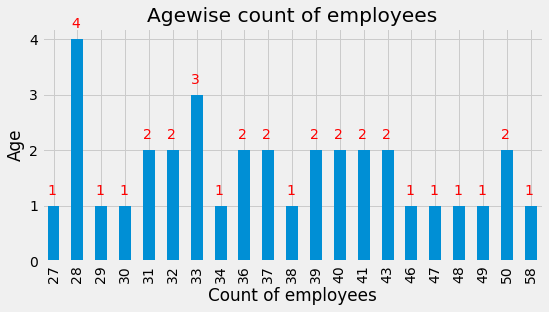## Educationwise Employee Count¶

The below graph shows that High School educated employees are higher than the rest.

In :
```edu_count = df.groupby(['Education_detail']).agg({'ID': pd.Series.nunique})
ax = edu_count.plot(kind='bar', figsize=(8,5), legend=False)
for i, v in enumerate(edu_count.values):
ax.text(i-.15, v + 1, str(v), color='red')
ax.set_xlabel('Education')
ax.set_ylabel('Count')
ax.set_title('Educationwise count of employees')
plt.show()
```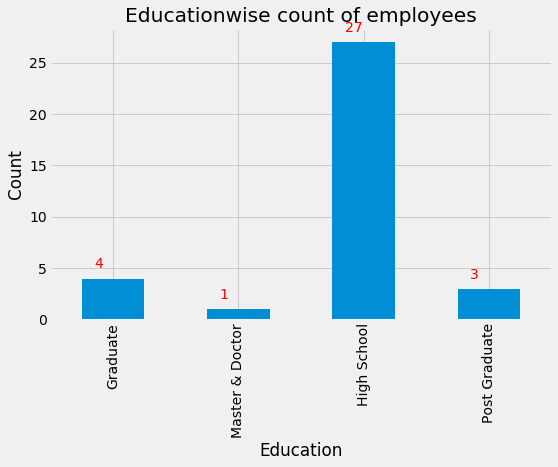I am not able to sort because of the categorical type of Education Detail column. You can see the below two commands where I try to use the observed flag, the results shifts. This seems to be a bug in pandas.

In :
```df.groupby('Education_detail').ID.agg({'ID': pd.Series.nunique})
```
```/home/rgrajan/anaconda3/lib/python3.7/site-packages/ipykernel_launcher.py:1: FutureWarning: using a dict on a Series for aggregation
is deprecated and will be removed in a future version
"""Entry point for launching an IPython kernel.
```
Out:
ID
Education_detail
Graduate 4
Master & Doctor 1
High School 27
Post Graduate 3
In :
```df.groupby('Education_detail', observed=True).ID.agg({'ID': pd.Series.nunique})
```
```/home/rgrajan/anaconda3/lib/python3.7/site-packages/ipykernel_launcher.py:1: FutureWarning: using a dict on a Series for aggregation
is deprecated and will be removed in a future version
"""Entry point for launching an IPython kernel.
```
Out:
ID
Education_detail
High School 4
Post Graduate 1
Graduate 27
Master & Doctor 3

## Average work load by Age¶

The work load seems to be same irrespective of the age.

In :
```age_work_sum = df.groupby('Age', as_index=False)[['Work load Averageperday']].mean()
ax = age_work_sum.plot(kind='bar', x='Age', figsize=(8,6), legend=False)
ax.set_ylabel('Work load average per day')
ax.set_title('Average work load per day by age')
plt.show()
```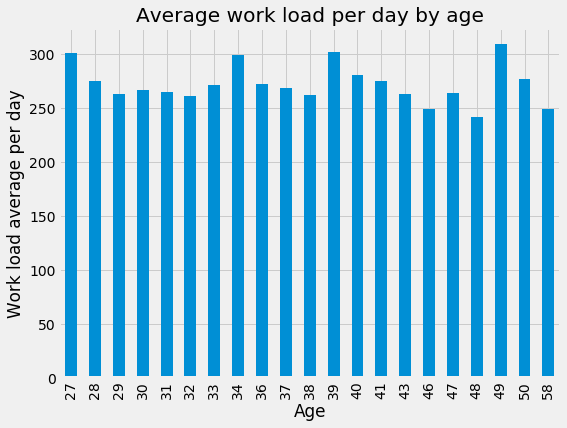## Average Absenteeism hours by Age¶

Absenteeism seems to be same across Age except for one age.

In :
```age_abs = df.groupby('Age')[['Absenteeism time in hours']].mean()
ax = age_abs.plot(kind='bar', figsize=(8,6), legend=False)
for i, v in enumerate(age_abs.values):
ax.text(i-.25, v + 1, str(np.int(np.round(v))), color='red')
ax.set_ylabel('Absenteeism time in hours')
ax.set_title('Average Absenteeism time in hours by age')
plt.show()
```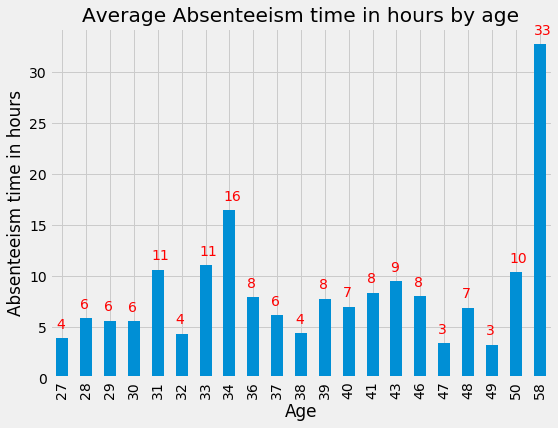## Average Absenteeism hours by Distance to work¶

The hypothesis i have was that if the distance to work increase the absenteeism hours will increase. But the below graph nullifies my hypothesis.

In :
```dis_abs = df.groupby('Distance from Residence to Work')[['Absenteeism time in hours']].mean()
ax = dis_abs.plot(kind='bar', figsize=(8,6), legend=False)
for i, v in enumerate(dis_abs.values):
ax.text(i-.25, v + 1, str(np.int(np.round(v))), color='red')
ax.set_xlabel('Distance from Residence to Work (km)')
ax.set_ylabel('Absenteeism time in hours')
ax.set_title('Average Absenteeism time in hours by distance')
plt.show()
```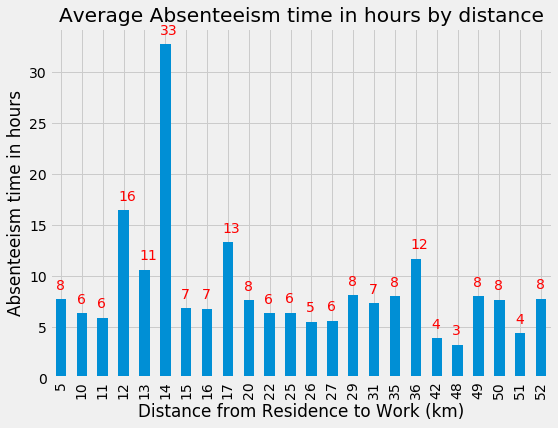## Average distance to work by Age¶

Another hypothesis I had was that higher age employees might stay closer to the office. It might be true till the Age 33, but the other values are not significant to compare.

In :
```age_dis = df.groupby('Age')[['Distance from Residence to Work']].mean()
ax = age_dis.plot(kind='bar', figsize=(8,6), legend=False)
for i, v in enumerate(age_dis.values):
ax.text(i-.25, v + 1, str(np.int(np.round(v))), color='red')
ax.set_ylabel('Distance from Residence to Work')
ax.set_title('Average Distance from Residence to Work by age')
plt.show()
```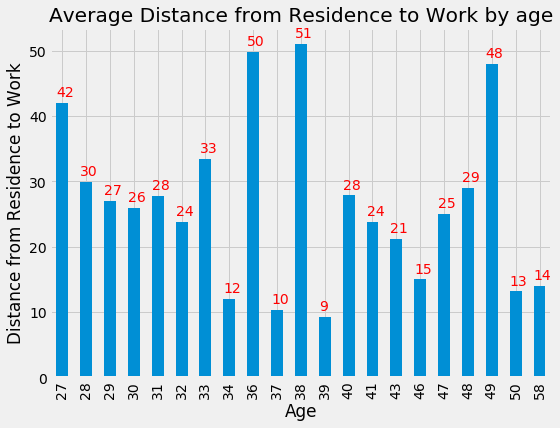## Average Transportation expense by Distance¶

The transportation expense is not increasing by distance but we don't have transport mode, so this is not helping us.

In :
```dis_exp = df.groupby('Distance from Residence to Work')[['Transportation expense']].mean()
ax = dis_exp.plot(kind='bar', figsize=(8,6), legend=False)
for i, v in enumerate(dis_exp.values):
ax.text(i-.45, v + 3, str(np.int(np.round(v))), color='red')
ax.set_ylabel('Transportation expense')
ax.set_title('Average Transportation expense by distance to work')
plt.show()
```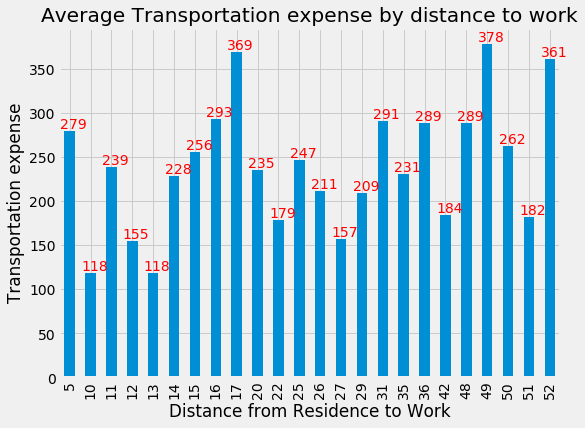## Pet & Son counts by Age¶

The below graph shows that the employees who has Son are mostly having a pet. This is interesting.

In :
```ax = df.groupby('Age')['Son', 'Pet'].sum().plot(figsize=(8,6))
ax.set_ylabel('Count')
ax.set_title('Count of Pet & Son by Age')
plt.show()
```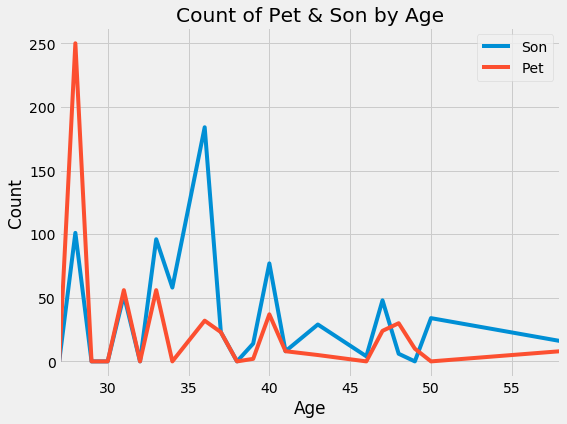## Smoker & Drinker Stats¶

How much percentage of Social Drinkers are Social Smokers also?

In :
```#emp_social = df.groupby(['ID', 'Social drinker', 'Social smoker'])[['Social drinker', 'Social smoker']].count()
emp_social = df.drop_duplicates(['ID', 'Social drinker', 'Social smoker'])[['ID', 'Social drinker', 'Social smoker']]
# % of Social drinker those are smokers
emp_social[emp_social['Social drinker']==True]['Social smoker'].mean()
```
Out:
`0.2222222222222222`

How much percentage of Social Smokers are Social Drinkers also?

In :
```# % of Social smokers are drinkers
emp_social[emp_social['Social smoker']==True]['Social drinker'].mean()
```
Out:
`0.5714285714285714`

## Absenteeism by Social habits¶

Looks like 60% of Social drinkers are absent and interestingly 32% of Non-smoker & Non-drinker are also absent.

In :
```drink_sum = df[(df['Social drinker'] == True) & (df['Social smoker']==False)]['Absenteeism time in hours'].sum()
smok_sum = df[(df['Social drinker'] == False) & (df['Social smoker']==True)]['Absenteeism time in hours'].sum()
drink_smok_sum = df[(df['Social drinker'] == True) & (df['Social smoker']==True)]['Absenteeism time in hours'].sum()
abs_sum = df[(df['Social drinker'] == False) & (df['Social smoker']==False)]['Absenteeism time in hours'].sum()
absen = [drink_sum, smok_sum, drink_smok_sum, abs_sum]
pie_labels = ['drinker', 'smoker', 'drinker & smoker', 'No drinker/smoker']
fig, ax = plt.subplots(figsize=(8, 6), subplot_kw=dict(aspect="equal"))

def func(pct, allvals):
absolute = int(pct/100.*np.sum(allvals))
return "{:.1f}%\n({:d})".format(pct, absolute)

wedges, texts, autotexts = ax.pie(absen, autopct=lambda pct: func(pct, absen), textprops=dict(color='w'))
ax.legend(wedges, pie_labels, title='Social Drinkers/Smokers', loc='right', bbox_to_anchor=(1, 0, 0.5, 1))
plt.setp(autotexts, size=10, weight="bold")
ax.set_title('Absenteeism by Social Drinkers/Smokers')
#plt.pie(absen, labels=pie_labels)
plt.show()
```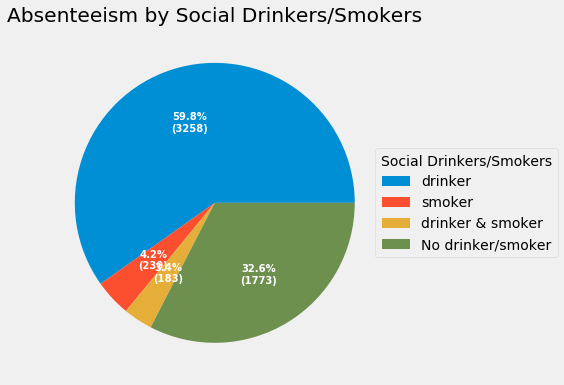## Absenteeism by Reason¶

The below table shows the highest Abseenteeism hours to lowest by Reason. The data is from courier company and the employees needs to deliver the packages and the Top 2 reason for absence shows that.
There is one hack to hide the index column.

In :
```reason_abs = df.groupby('reason_text', as_index=False)['Absenteeism time in hours'].sum()
with pd.option_context('display.max_colwidth', -1):
display(reason_abs.sort_values('Absenteeism time in hours', ascending=False).style.hide_index())
```
reason_text Absenteeism time in hours
Diseases of the musculoskeletal system and connective tissue 842
Injury, poisoning and certain other consequences of external causes 729
Medical consultation 424
Dental consultation 335
Unknown 320
Diseases of the digestive system 297
Patient follow-up 293
Diseases of the respiratory system 276
Unjustified absence 240
Symptoms, signs and abnormal clinical and laboratory findings, not elsewhere classified 217
Diseases of the skin and subcutaneous tissue 187
Certain infectious and parasitic diseases 182
Diseases of the nervous system 171
Diseases of the circulatory system 168
Diseases of the genitourinary system 167
Physiotherapy 160
Diseases of the eye and adnexa 150
Laboratory examination 108
Factors influencing health status and contact with health services 35
Diseases of the ear and mastoid process 32
Neoplasms 24
Blood donation 24
Mental and behavioural disorders 19
Pregnancy, childbirth and the puerperium 16
Endocrine, nutritional and metabolic diseases 9
Diseases of the blood and blood-forming organs and certain disorders involving the immune mechanism 8
Congenital malformations, deformations and chromosomal abnormalities 8
Certain conditions originating in the perinatal period 6

## Absenteeism hours by Seasons¶

Looks like winter has highest Absenteeism.

In :
```season_abs = df.groupby('season_name')['Absenteeism time in hours'].sum()
ax = season_abs.plot(kind='bar', figsize=(6,4), legend=False)
for i, v in enumerate(season_abs.values):
ax.text(i-.17, v + 35, str(np.int(np.round(v))), color='red')
ax.set_xlabel('Seasons')
ax.set_ylabel('Sum of Absenteeism hours')
ax.set_title('Sum of Absenteeism hours by Seasons')
plt.show()
```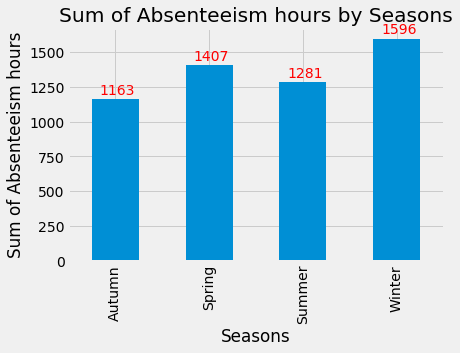## Absenteeism hours by Month¶

Getting to detail from Seasons to Month, Looks like March & July has the highest number of Absenteeism hours.

In :
```month_abs = df.groupby('month_name')['Absenteeism time in hours'].sum()
ax = month_abs.plot(kind='bar', figsize=(8,6), legend=False)
for i, v in enumerate(month_abs.values):
ax.text(i-0.2, v + 25, str(np.int(np.round(v))), color='red')
ax.set_xlabel('Month')
ax.set_ylabel('Sum of Absenteeism hours')
ax.set_title('Sum of Absenteeism hours by Month')
plt.show()
```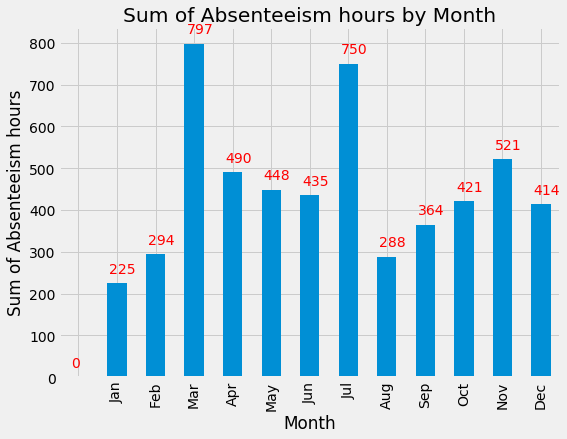### March month Absenteeism hours by Reason¶

Let us check the March month reason.

In :
```mar_abs = df[df['Month of absence']==3].groupby('reason_text', as_index=False)['Absenteeism time in hours'].sum()
with pd.option_context('display.max_colwidth', -1):
display(mar_abs.sort_values('Absenteeism time in hours', ascending=False).style.hide_index())
```
reason_text Absenteeism time in hours
Injury, poisoning and certain other consequences of external causes 147
Diseases of the musculoskeletal system and connective tissue 136
Diseases of the skin and subcutaneous tissue 112
Diseases of the digestive system 72
Patient follow-up 56
Dental consultation 43
Physiotherapy 41
Diseases of the genitourinary system 33
Unknown 32
Medical consultation 28
Unjustified absence 16
Certain infectious and parasitic diseases 16
Laboratory examination 13
Congenital malformations, deformations and chromosomal abnormalities 8
Factors influencing health status and contact with health services 8
Diseases of the nervous system 8
Diseases of the eye and adnexa 8
Diseases of the ear and mastoid process 8
Symptoms, signs and abnormal clinical and laboratory findings, not elsewhere classified 8
Diseases of the respiratory system 4

### July month Absenteeism hours by Reason¶

Looks like the March month & July month reasons are not matching.

In :
```jul_abs = df[df['Month of absence']==7].groupby('reason_text', as_index=False)['Absenteeism time in hours'].sum()
with pd.option_context('display.max_colwidth', -1):
display(jul_abs.sort_values('Absenteeism time in hours', ascending=False).style.hide_index())
```
reason_text Absenteeism time in hours
Diseases of the nervous system 128
Diseases of the circulatory system 112
Injury, poisoning and certain other consequences of external causes 98
Diseases of the musculoskeletal system and connective tissue 88
Patient follow-up 72
Unjustified absence 56
Certain infectious and parasitic diseases 40
Medical consultation 24
Diseases of the digestive system 21
Dental consultation 18
Laboratory examination 17
Diseases of the genitourinary system 16
Unknown 16
Diseases of the eye and adnexa 12
Factors influencing health status and contact with health services 8
Mental and behavioural disorders 8
Pregnancy, childbirth and the puerperium 8
Symptoms, signs and abnormal clinical and laboratory findings, not elsewhere classified 8

# Feature Extraction¶

Tired of EDA? Let us get into Feature Engineering.

In :
```df_features = df[['Reason for absence', 'Month of absence', 'Day of the week', 'Seasons', 'Transportation expense',
'Distance from Residence to Work', 'Service time', 'Age', 'Work load Averageperday', 'Hit target',
'Disciplinary failure', 'Education', 'Son', 'Social drinker', 'Social smoker', 'Pet', 'Weight',
'Height', 'Body mass index' ]]
```

The below graph shows the spearman ranking correleation between the features. Using this dendogram we can remove redundant features. But this dataset didn't have any redundant features. I learnt this from Jeremy Howard

In :
```corr = np.round(scipy.stats.spearmanr(df_features).correlation, 4)
corr_condensed = hc.distance.squareform(1-corr)
z = hc.linkage(corr_condensed, method='average')
fig = plt.figure(figsize=(16,10))
dendrogram = hc.dendrogram(z, labels=df_features.columns, orientation='left', leaf_font_size = 16)
plt.show()
```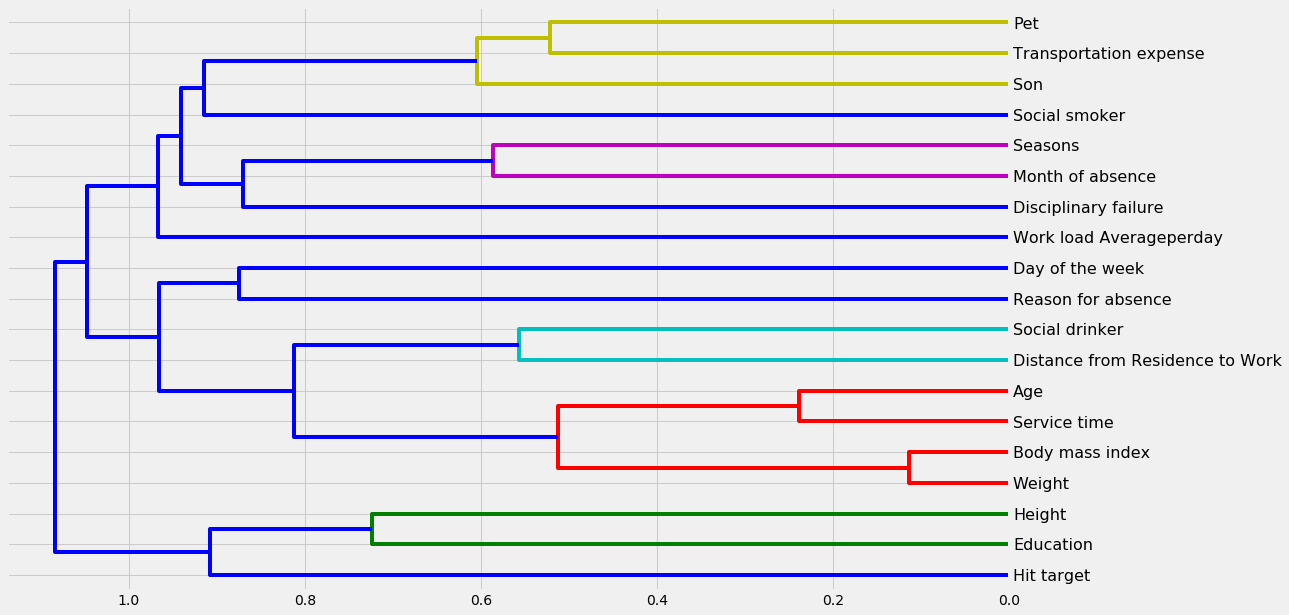# Prediction¶

Even though this dataset is categorized as Time Series, i am not able to create Period Index with pandas. So I have decided to use other algorithms instead of ARIMA.

## Train, Test Split¶

This is common standard to create a validation set to verify the model. We are using sklearn inbuilt methods to split the data. I have decided to try Random Forest for this dataset.

In :
```X = df_features
y = df['Absenteeism time in hours']
X_train, X_test, y_train, y_test = train_test_split(X, y, test_size=0.3, random_state=42)
```

## ML Model Creation¶

This is a regression problem as we have to predict the Absenteeism hours from the features. Let us use Random Forest Regressor to create the model.

In :
```model = RandomForestRegressor(n_estimators=1000, random_state=0, oob_score=True, n_jobs=-1)
model.fit(X_train,y_train)
y_pred = model.predict(X_test)
```

## Model Metrics¶

This is otherwise called evaluation of the model. Here we are comparing the predicted values with data we already have. This will give us the accuracy of the model.

In :
```test_score = r2_score(y_test, y_pred)
spearman = spearmanr(y_test, y_pred)
pearson = pearsonr(y_test, y_pred)
rmse_test = MSE(y_test, y_pred) ** (1/2)

print(f'Out-of-bag score : {model.oob_score_:>5.3}')
print(f'Test data R-2 score: {test_score:>5.3}')
print(f'Test set of RMSE : {rmse_test:.2f}')
print(f'Test data Spearman correlation: {spearman:.3}')
print(f'Test data Pearson correlation: {pearson:.3}')
```
```Out-of-bag score : -0.0335
Test data R-2 score: 0.0224
Test set of RMSE : 12.87
Test data Spearman correlation: 0.647
Test data Pearson correlation: 0.302
```

The negative out-of-bag score is the worst model you can ever see in the Random Forest. R2 score is nearly zero the model is not predicting anything.

## Feature Importance¶

This is one way of interpreting the Random Forest model.

In :
```ax = (pd.Series(model.feature_importances_, index=X.columns)
.nlargest(19)
.plot(kind='barh', figsize=(8,6), color='lightgreen'))
plt.show()
```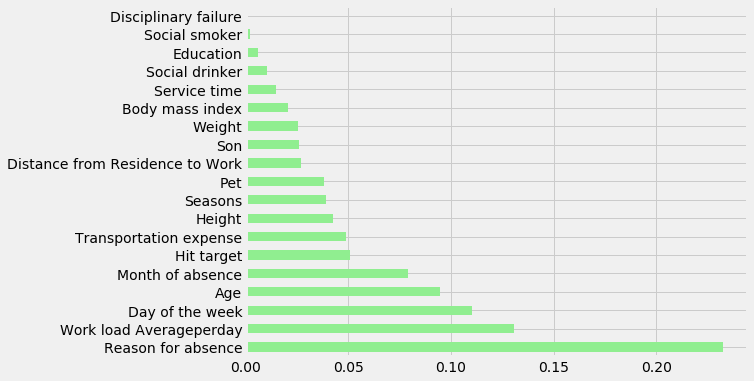## Classification¶

This is the challenge in the small dataset. Instead of a regression, why don't we change the target variable as Classification? If this gives better results then there is no issue, isn't it?

The target variable is Absenteeism in hours. Let us change to classification, so the multiclass variable is <4, 8, 16, 16+. This is just random assumption that employee absence can be half day, full day, 2 days or more...

In :
```abs_bins = [0, 4, 8, 16, np.inf]
abs_names = ['<4', '8', '16', '16+']
df['abs_range'] = pd.cut(df['Absenteeism time in hours'], abs_bins, labels=abs_names)
```

## ML Model Creation¶

Let us split the data and create Random Forest Classifier

In :
```X = df_features
y = df['abs_range']
X_train, X_test, y_train, y_test = train_test_split(X, y, test_size=0.3, random_state=42)
```
In :
```model = RandomForestClassifier(n_estimators=100, random_state=0, oob_score=True, n_jobs=-1)
model.fit(X_train,y_train)
y_pred = model.predict(X_test)
```

## Model Metrics¶

Let us evaluate the model

In :
```print("Accuracy:",metrics.accuracy_score(y_test, y_pred))
```
```Accuracy: 0.7342342342342343
```
In :
```print(classification_report(y_test, y_pred))
```
```              precision    recall  f1-score   support

16       0.00      0.00      0.00         3
16+       0.33      0.18      0.24        11
8       0.66      0.64      0.65        78
<4       0.80      0.85      0.83       130

micro avg       0.73      0.73      0.73       222
macro avg       0.45      0.42      0.43       222
weighted avg       0.72      0.73      0.72       222

```

The above accuracy report is better compare to the earlier regession model. The micro F1 score 0.73 is good compare to negative out-of-bag score.

## Feature Importance¶

This is one way of interpreting the Random Forest Model and we can see the contributions from the features. This is at high level we can understand the importance of features.

In :
```ax = (pd.Series(model.feature_importances_, index=X.columns)
.nlargest(19)
.plot(kind='barh', figsize=(8,6), color='lightgreen'))
plt.show()
```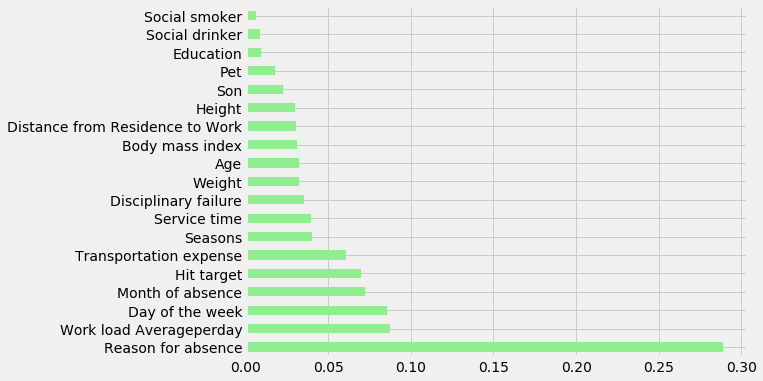## Feature Engineering¶

If the Absenteeism in hours can be in range, I wanted to check whether model accuracy changes if any one of feature changes. We have seen earlier during EDA that Age is so discrete. Let us convert the Age to Age Range.

In :
```bins = [25, 35, 45, 55, np.inf]
names = [25, 35, 45, 55]
df['age_range'] = pd.cut(df['Age'], bins, labels=names)
```
In :
```X = df[['Reason for absence', 'Month of absence', 'Day of the week', 'Seasons', 'Transportation expense',
'Distance from Residence to Work', 'Service time', 'age_range', 'Work load Averageperday', 'Hit target',
'Disciplinary failure', 'Education', 'Son', 'Social drinker', 'Social smoker', 'Pet', 'Weight',
'Height', 'Body mass index' ]]
y = df['abs_range']
X_train, X_test, y_train, y_test = train_test_split(X, y, test_size=0.3, random_state=42)
```
In :
```model = RandomForestClassifier(n_estimators=100, random_state=0, oob_score=True, n_jobs=-1)
model.fit(X_train,y_train)
y_pred = model.predict(X_test)
```
In :
```print("Accuracy:",metrics.accuracy_score(y_test, y_pred))
```
```Accuracy: 0.7432432432432432
```
In :
```print(classification_report(y_test, y_pred))
```
```              precision    recall  f1-score   support

16       0.00      0.00      0.00         3
16+       0.33      0.18      0.24        11
8       0.68      0.63      0.65        78
<4       0.80      0.88      0.84       130

micro avg       0.74      0.74      0.74       222
macro avg       0.45      0.42      0.43       222
weighted avg       0.72      0.74      0.73       222

```

The accuracy is improving. This shows that there is no end to Feature Engineering. And it is evolving process and we have to do better feature engineering to get the better results.

# Hyper Parameter tuning with Cross Validation¶

Hyper parameter tuning is a process is to change the parameters of the model and identify which parameter gives us the better accuracy. The cross validation in Grid & Random Search helps us to use Stratified K-fold to create validation set within trained data. The CV value 5 or above uses Stratified K-fold.

In :
```from sklearn.model_selection import RandomizedSearchCV
n_estimators = [int(x) for x in np.linspace(start=100, stop = 2000, num = 20)]
max_features = ['auto', 'sqrt']
max_depth = [int(x) for x in np.linspace(10, 110, num = 11)]
max_depth.append(None)
min_samples_split = [2, 5, 10]
min_sample_leaf = [1, 2, 4]
bootstrap = [True, False]

random_grid = {'n_estimators' : n_estimators,
'max_features' : max_features,
'max_depth' : max_depth,
'min_samples_split' : min_samples_split,
'min_samples_leaf': min_sample_leaf,
'bootstrap' : bootstrap
}

rf = RandomForestClassifier()
rf_random = RandomizedSearchCV(estimator = rf, param_distributions = random_grid, n_iter = 100, cv=5, error_score= np.nan,
verbose=2, random_state=42, n_jobs = -1)
rf_random.fit(X_train, y_train)
```
```Fitting 5 folds for each of 100 candidates, totalling 500 fits
```
```[Parallel(n_jobs=-1)]: Using backend LokyBackend with 4 concurrent workers.
[Parallel(n_jobs=-1)]: Done  33 tasks      | elapsed:   17.2s
[Parallel(n_jobs=-1)]: Done 154 tasks      | elapsed:  1.1min
[Parallel(n_jobs=-1)]: Done 357 tasks      | elapsed:  2.4min
[Parallel(n_jobs=-1)]: Done 500 out of 500 | elapsed:  3.4min finished
/home/rgrajan/anaconda3/lib/python3.7/site-packages/sklearn/model_selection/_search.py:841: DeprecationWarning: The default of the `iid` parameter will change from True to False in version 0.22 and will be removed in 0.24. This will change numeric results when test-set sizes are unequal.
DeprecationWarning)
```
Out:
```RandomizedSearchCV(cv=5, error_score=nan,
estimator=RandomForestClassifier(bootstrap=True, class_weight=None, criterion='gini',
max_depth=None, max_features='auto', max_leaf_nodes=None,
min_impurity_decrease=0.0, min_impurity_split=None,
min_samples_leaf=1, min_samples_split=2,
min_weight_fraction_leaf=0.0, n_estimators='warn', n_jobs=None,
oob_score=False, random_state=None, verbose=0,
warm_start=False),
fit_params=None, iid='warn', n_iter=100, n_jobs=-1,
param_distributions={'n_estimators': [100, 200, 300, 400, 500, 600, 700, 800, 900, 1000, 1100, 1200, 1300, 1400, 1500, 1600, 1700, 1800, 1900, 2000], 'max_features': ['auto', 'sqrt'], 'max_depth': [10, 20, 30, 40, 50, 60, 70, 80, 90, 100, 110, None], 'min_samples_split': [2, 5, 10], 'min_samples_leaf': [1, 2, 4], 'bootstrap': [True, False]},
pre_dispatch='2*n_jobs', random_state=42, refit=True,
return_train_score='warn', scoring=None, verbose=2)```

The below will provide us the best parameters from the Random Search CV.

In :
```rf_random.best_params_
```
Out:
```{'n_estimators': 300,
'min_samples_split': 10,
'min_samples_leaf': 1,
'max_features': 'sqrt',
'max_depth': 10,
'bootstrap': True}```

Let us use the above model to predict & evaluate.

In :
```from sklearn.ensemble import RandomForestClassifier
model_random = rf_random.best_estimator_
model_random.fit(X_train, y_train)
predictions_random = model_random.predict(X_test)
```
In :
```print("Accuracy:",metrics.accuracy_score(y_test, predictions_random))
```
```Accuracy: 0.7432432432432432
```
In :
```print(classification_report(y_test, predictions_random))
```
```              precision    recall  f1-score   support

16       0.00      0.00      0.00         3
16+       1.00      0.09      0.17        11
8       0.66      0.71      0.68        78
<4       0.79      0.84      0.81       130

micro avg       0.74      0.74      0.74       222
macro avg       0.61      0.41      0.42       222
weighted avg       0.74      0.74      0.72       222

```
```/home/rgrajan/anaconda3/lib/python3.7/site-packages/sklearn/metrics/classification.py:1143: UndefinedMetricWarning: Precision and F-score are ill-defined and being set to 0.0 in labels with no predicted samples.
'precision', 'predicted', average, warn_for)
/home/rgrajan/anaconda3/lib/python3.7/site-packages/sklearn/metrics/classification.py:1143: UndefinedMetricWarning: Precision and F-score are ill-defined and being set to 0.0 in labels with no predicted samples.
'precision', 'predicted', average, warn_for)
/home/rgrajan/anaconda3/lib/python3.7/site-packages/sklearn/metrics/classification.py:1143: UndefinedMetricWarning: Precision and F-score are ill-defined and being set to 0.0 in labels with no predicted samples.
'precision', 'predicted', average, warn_for)
```

The above warning shows that the data is not available for few classes during the cross validation. I don't want to suppress the warnings and also you can see the micro-F1 score below without the warning.

In :
```metrics.f1_score(y_test, predictions_random, average='micro')
```
Out:
`0.7432432432432431`

Let us check the train & test data for each classification.

In :
```Counter(y_train)
```
Out:
`Counter({'8': 178, '<4': 288, '16+': 33, '16': 16})`
In :
```Counter(y_test)
```
Out:
`Counter({'<4': 130, '8': 78, '16+': 11, '16': 3})`

The above value shows that there are less values in the test/validation set and the warning is obvious.

The random search gives us the range of the values and using that We can do the Grid Search to tune the model with exact parameters.

In :
```from sklearn.model_selection import GridSearchCV

param_grid = {
'bootstrap' : [True],
'max_depth' : [8, 10, 12, 14],
'max_features' : ['sqrt'],
'min_samples_leaf' : [1, 3, 4],
'min_samples_split' : [7, 10, 12],
'n_estimators' : [250, 275, 300, 325]

}

rf = RandomForestClassifier()
grid_search = GridSearchCV(estimator = rf, param_grid = param_grid, cv=5, n_jobs = -1, error_score=np.nan, verbose = 2)

grid_search.fit(X_train, y_train)
```
```Fitting 5 folds for each of 144 candidates, totalling 720 fits
```
```[Parallel(n_jobs=-1)]: Using backend LokyBackend with 4 concurrent workers.
[Parallel(n_jobs=-1)]: Done  33 tasks      | elapsed:    4.1s
[Parallel(n_jobs=-1)]: Done 154 tasks      | elapsed:   18.2s
[Parallel(n_jobs=-1)]: Done 357 tasks      | elapsed:   41.9s
[Parallel(n_jobs=-1)]: Done 640 tasks      | elapsed:  1.3min
[Parallel(n_jobs=-1)]: Done 720 out of 720 | elapsed:  1.5min finished
/home/rgrajan/anaconda3/lib/python3.7/site-packages/sklearn/model_selection/_search.py:841: DeprecationWarning: The default of the `iid` parameter will change from True to False in version 0.22 and will be removed in 0.24. This will change numeric results when test-set sizes are unequal.
DeprecationWarning)
```
Out:
```GridSearchCV(cv=5, error_score=nan,
estimator=RandomForestClassifier(bootstrap=True, class_weight=None, criterion='gini',
max_depth=None, max_features='auto', max_leaf_nodes=None,
min_impurity_decrease=0.0, min_impurity_split=None,
min_samples_leaf=1, min_samples_split=2,
min_weight_fraction_leaf=0.0, n_estimators='warn', n_jobs=None,
oob_score=False, random_state=None, verbose=0,
warm_start=False),
fit_params=None, iid='warn', n_jobs=-1,
param_grid={'bootstrap': [True], 'max_depth': [8, 10, 12, 14], 'max_features': ['sqrt'], 'min_samples_leaf': [1, 3, 4], 'min_samples_split': [7, 10, 12], 'n_estimators': [250, 275, 300, 325]},
pre_dispatch='2*n_jobs', refit=True, return_train_score='warn',
scoring=None, verbose=2)```
In :
```grid_search.best_params_
```
Out:
```{'bootstrap': True,
'max_depth': 12,
'max_features': 'sqrt',
'min_samples_leaf': 4,
'min_samples_split': 7,
'n_estimators': 275}```
In :
```from sklearn.ensemble import RandomForestClassifier
model_grid = grid_search.best_estimator_
model_grid.fit(X_train, y_train)
predictions_grid = model_grid.predict(X_test)
```
In :
```print("Accuracy:",metrics.accuracy_score(y_test, predictions_grid))
```
```Accuracy: 0.7477477477477478
```
In :
```print(classification_report(y_test, predictions_grid))
```
```              precision    recall  f1-score   support

16       0.00      0.00      0.00         3
16+       1.00      0.09      0.17        11
8       0.68      0.69      0.68        78
<4       0.79      0.85      0.82       130

micro avg       0.75      0.75      0.75       222
macro avg       0.62      0.41      0.42       222
weighted avg       0.75      0.75      0.73       222

```
```/home/rgrajan/anaconda3/lib/python3.7/site-packages/sklearn/metrics/classification.py:1143: UndefinedMetricWarning: Precision and F-score are ill-defined and being set to 0.0 in labels with no predicted samples.
'precision', 'predicted', average, warn_for)
/home/rgrajan/anaconda3/lib/python3.7/site-packages/sklearn/metrics/classification.py:1143: UndefinedMetricWarning: Precision and F-score are ill-defined and being set to 0.0 in labels with no predicted samples.
'precision', 'predicted', average, warn_for)
/home/rgrajan/anaconda3/lib/python3.7/site-packages/sklearn/metrics/classification.py:1143: UndefinedMetricWarning: Precision and F-score are ill-defined and being set to 0.0 in labels with no predicted samples.
'precision', 'predicted', average, warn_for)
```

There is minor change in the accuracy but the model is improving, so it is reliable.

# Interpreting the Model¶

The most common problem we have is to justify the predictions. Why the model has predicted in a way? otherwise it is alwo known as blackbox.

This challenge can be solved easily in the Random Forest by the beautiful python package TreeInterpreter

Let us take one row and try to predict & interpret.

In :
```row = X_test.values[None, 0]
row
```
Out:
```array([[27, 4, 6, 3, 179, 26, 9, 25, 246.28799999999998, 91, False, 3, 0,
False, False, 0, 56, 171, 19]], dtype=object)```

Let us predict using Tree Interpreter for the above feature. The Tree Interpreter provides us the prediction, bias of the trainset & contributions to prediction for each feature. I have used the initial model and we can use any model.

In :
```prediction, bias, contributions = ti.predict(model, row)
```

Let us check the prediction & bias values.

In :
```prediction, bias
```
Out:
```(array([0.02, 0.  , 0.03, 0.95]),
array([0.03097087, 0.06580583, 0.34458252, 0.55864078]))```

To check the prediction of the Tree Interpreter, let us predict for the same feature using Random Forest model. Here we are using prediction probability to compare the values.

In :
```print(model.predict_proba(row))
```
```[[0.02 0.   0.03 0.95]]
```

The Tree interpreter values and the model's prediction probability matches and the 4th class has the highest value. Now let us check the classess from the model.

In :
```model.classes_
```
Out:
`array(['16', '16+', '8', '<4'], dtype=object)`

As per the classes above, the prediction is <4 for the selected row. Let us check that also.

In :
```print(model.predict(row))
```
```['<4']
```

Most importantly we have to see the contributions for each feature. The below list clearly shows the contributions for all the 4 classes.

In :
```for c, feature in zip(contributions,
X.columns):
print (feature, c)
```
```Reason for absence [-0.01397725 -0.04777173 -0.18694341  0.24869239]
Month of absence [-0.0102317   0.00053675 -0.00806588  0.01776083]
Day of the week [-0.00053484 -0.00368252  0.00435736 -0.00013999]
Seasons [-0.00308563  0.00031326 -0.00800344  0.01077581]
Transportation expense [-0.00110594 -0.00493542 -0.04178329  0.04782466]
Distance from Residence to Work [ 0.00037238 -0.00110435 -0.01242714  0.01315911]
Service time [ 0.00088356 -0.00111264 -0.00818415  0.00841323]
age_range [ 0.00100485  0.00052618  0.00343277 -0.00496381]
Work load Averageperday [-0.00873143 -0.00174223 -0.01200415  0.02247781]
Hit target [-0.00301136 -0.00071768  0.00511491 -0.00138587]
Disciplinary failure [ 0.00053817  0.00108183 -0.01358447  0.01196448]
Education [ 0.00472451 -0.00034883  0.00028677 -0.00466245]
Son [-0.00083389 -0.00181832 -0.01033848  0.01299068]
Social drinker [-7.72350629e-05 -2.57300017e-03 -5.13677811e-03  7.78701335e-03]
Social smoker [-0.00037394  0.         -0.00119288  0.00156682]
Pet [-0.00027488  0.00015285 -0.00282086  0.0029429 ]
Weight [ 0.0101779  -0.00134286 -0.00337288 -0.00546216]
Height [ 0.00101011 -0.00157102 -0.01631335  0.01687427]
Body mass index [ 0.01255573  0.00030392  0.00239684 -0.01525649]
```

Let us sum the contributions for this row.

In :
```print(contributions.sum(axis=1))
```
```[[-0.01097087 -0.06580583 -0.31458252  0.39135922]]
```

We have the bias from the trainset, let us add the above contributions to the bias.

In :
```with np.printoptions(precision=3, suppress=True):
print(contributions.sum(axis=1) + bias)
```
```[[ 0.02 -0.    0.03  0.95]]
```

The above matches with earlier prediction probabilities from the model. Now we know exactly the why the model has predicted the 4th class <4.

# Conclusion¶

• Even if the dataset is small we should be able to analyze and predict.
• The Random Forest model is easier to interpret and we don't need to worry about answering the reasoning.